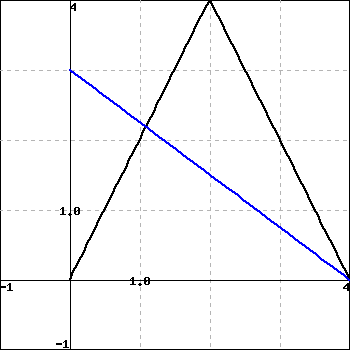Use the graph below to find exact values of the indicated derivatives, or state that they do not exist. If a derivative does not exist, enter dne in the answer blank. The graph of $f(x)$ is black and has a sharp corner at $x = 2$. The graph of $g(x)$ is blue.Let $h(x) = f(g(x))$. Find

A. $h'(1) =$
B. $h'(2) =$
C. $h'(3) =$

(Enter dne for any derivative that does not exist.)